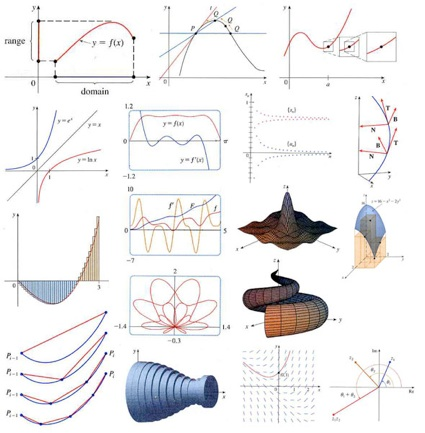# 61. Learn Advanced Calculus & Analytic Geometry

Completed April, 1998 @ Lacombe, ABI haphazardly completed this goal. I thought there was more to this, but according to Math Master Kydd, it so happens that the courses I took in university is about it. I always did my homework on recycled paper from the library.
I had the great opportunity to teach Math 31 for five years at Bearspaw Christian School. It covers about 3/4 of first semester university calculus. I’ve pretty good at those chapters now.

Topics include:

• Limits and Rates of Change
• Derivatives
• Inverse Functions
• Mean Value Theorem and Curve Sketching
• Integrals
• Applications of Integration
• Techniques of Integration
• Further Applications of Integration
• Parametric Equations and Polar Coordinates
• Infinite Sequences and Series
• Three-Dimensional Analytic Geometry and Vectors
• Partial Derivatives
• Multiple Integrals
• Vector Calculus
• Differential Equations
• Linear Algebra and its Applications